# AP Calculus AB : Definite integral as a limit of Riemann sums

## Example Questions

### Example Question #1 : Definite Integral As A Limit Of Riemann Sums

True or False: Ifis a negative-valued function for all,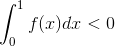False

True

True

Explanation:

This is true. Sinceis negative-valued, its graph is below the-axis, and the Riemann sums used to evaluate the area betweenand the-axis have a negative value for height.

### Example Question #1 : Definite Integral As A Limit Of Riemann Sumsis a continuous function on the interval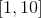and is differentiable on the open interval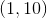.  If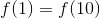, then which of the following statements MUST be true: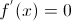over the interval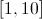.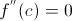at some point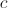, where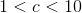.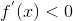over the interval.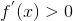over the interval.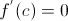at some point, where.at some point, where.

Explanation:

According to Rolle's Theorem, if a function is continuous over a closed interval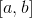and differentiable on the open interval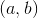, and if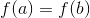, then there has to be some valuesuch that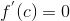, where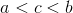.  To put this another way, if a function is continuous and differentiable over a certain interval, and if the value of the function is the same at both endpoints of that interval, then at some point in between those endpoints, the function is going to have a slope of zero (i.e. its first derivative will be zero).  This does not apply to the second derivative, nor does it require that the slope of the first derivative be zero over the entire interval.

### Example Question #1 : Definite Integral As A Limit Of Riemann Sums

You may use one or both of the following summation formulas: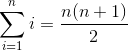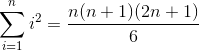Express the following definite integral as a limit of Riemman Sums. Then evaluate the integral by evaluating the limit.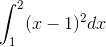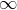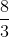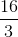Explanation:

First, let's remind ourselves what the formula for Riemann Sums is. Written in Summation form, it is: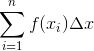when, the number of subintervals, is small, the area calculated by the Riemann Sums is not very accurate. However, as we increase the number of subintervals, the approximation becomes closer and closer to the exact area under the function. "Closer and closer" is a concept from Limits. So if we find the limit of the Riemann sum formula, with n approaching infinity, the result is the exact area. This is the essence of the Definite integral definition. What it effectively tells us to do is stick a limit on the Riemann sums formula to get: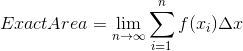To use this formula, we need to do three things:

(1) we need to find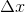(2) we need to develop a formula for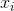(3) we need to plug thatinto the function inside the given integral.

To find, we use the same formula from Riemann sums.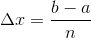, whereandare the upper and lower bounds from the definite integral. For our integral,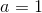and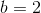. Since we are not stating a specific number of subintervals, we leaveas it is. Nothing gets plugged into it.

Plugging inand, we get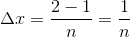Now we have. Next we find a formula for, which by the way represents the right endpoint of the "i-th" subinterval. We will revisit that sentence shortly to clarify.

First, we know that the left endpoint of the 1st subinterval is, and that this subinterval has a width of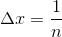. So to get the right endpoint of the 1st subinterval, we just add that width to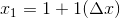To get the 2nd right endpoint, we just add a 2nd.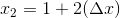to get the "i-th" right endpoint, we just add"i" times.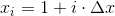This is the "i-th" concept. Now if we wanted the 50th rigth endpoint, we would just plug 50 in for "i". Fortunately, we don't need to.

Now we haveand we have a formula for. Next we will find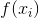by plugging our formula in to the function from inside our integral. In other words our function is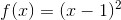.

Plugging in to this function we get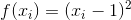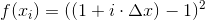Recall that we determined. Pluggin that in, we get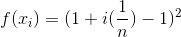To simplify, we will combine the 1 and -1 to cancel them,  and then apply the square power to what is left.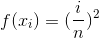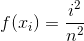Now we have all the pieces of the Limit of Riemann sums formula. Let's plug them in.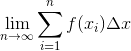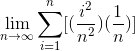To evaluate the limit, we must first find the sum. To do this, we must realize that we can pull any common factors out of the summation, as long as they are constant with respect to the summation index. In other words, we can pull out any variable that is not an. This means we can write the's on the left of the sum, while still writing them inside the limit,

like this: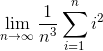By passing the's to the left of the sum, we have matched our sum to one of the provided sum formulas, specifically:This means we can evaluate the sum by replacing it using the above formula.

Doing this, we get: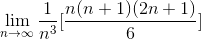Now we can start evaluating the limit.

Lets start by reducing the singlefrom top and with one from the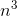on bottom.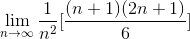Now we will multiply our the two factors on top, and also multiply the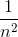through the other fraction.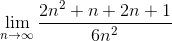Combining like terms, we get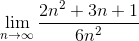Since the denominator is a single term, we can split the big fraction into three individual fractions, and reduce each one.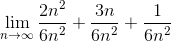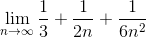Remember that the limit of a constant is equal to that constant. Also remember when evaluating limits at infinity, a constant divided by a variable equals zero.

Applying these, we get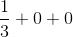This gives us the correct answer of### All AP Calculus AB Resources